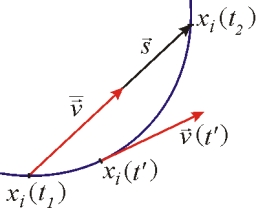# Motorcyclist 8671

The motorcyclist was riding:

a) the first half of the driving time at a speed of 30 km/h, the second half of the time at a speed of 60 km/h,
b) the track's first half at a speed of 30 km/h and the second half at a speed of 60 km/h.

Determine its average speed.

v1 =  40 km/h
v2 =  40 km/h

### Step-by-step explanation:Did you find an error or inaccuracy? Feel free to write us. Thank you!

Tips for related online calculators
Looking for calculator of harmonic mean?
Looking for a statistical calculator?
Do you want to convert velocity (speed) units?